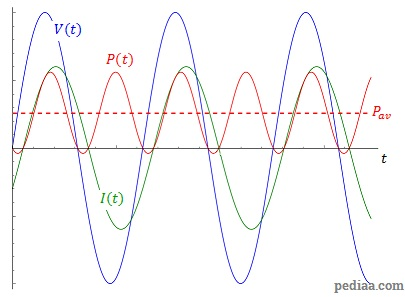# Difference Between AC and DC Power

## Main Difference – AC vs. DC Power

Components connected to AC as well as DC circuits dissipate power. The terms “AC Power” and “DC Power” refer to the power dissipated in these two different types of circuits. At a fundamental level, the same concepts are used to calculate power in both types of circuits. However, because the direction of current is always changing in AC circuits, the power dissipated also changes periodically. The main difference between AC and DC power is that, in DC circuits, the power dissipated remains a constant while, in AC circuits, the power dissipated varies periodically.

## What is DC Power

DC current refers to a current formed by electrons flowing continuously in one direction. As the electrons flow through various components in the circuit, they lose their electrical energy. DC power refers to the amount of energy  dissipated per second by a coulomb of electrons, as they travel between two points in a circuit. In a DC circuit, if a component has a potential difference$V$ across it and a current$I$ flowing through it, and if the component’s resistance is$R$, then the power dissipated by the component is given by:$P=VI=\frac{V^2}{R}=I^2R$

If the DC current is steady, then the power dissipated by the component will also be nearly constant (we are assuming that the temperature and other physical factors that affect power dissipation does not change). Most everyday components, including mobile phones and personal computers, use DC current to operate.

## What is AC Power

AC current refers to currents formed by electrons moving back and forth, set up by a terminal that has an oscillating voltage. During the cycle of back-forth motion, electrons periodically speed up, slow down, and come to a momentary stop. Therefore, the current is also changing periodically. The instantaneous power$P(t)$ refers to the amount of power dissipated between two points in the circuit/by a component at any given time. It is given by:$P(t)=V(t)I(t)$

where$V(t)$ and$I(t)$ are the potential difference and current at that time.

However, because$V(t)$ and$I(t)$ are always changing, instantaneous power is also constantly changing. The average power is a much more useful concept for components connected to AC circuits. When the time taken to complete a full oscillation by electrons (i.e. their period) is given by$T$, average power can be calculated as:$P_{av}=\frac{1}{T}\int_{0}^{T}P(t)\mathrm dt$

Suppose potential difference$V(t)$ across the component varies sinusoidally, and that the current$I(t)$ lags behind the voltage by a phase angle$\phi$. Then we can show that the average power can be given by:$P_{av}=V_{rms}I_{rms}\mathrm{cos} \phi$

Here,$V_{rms}$ and$I_{rms}$ refer to the root mean square values of voltage and current respectively, i.e. when the maximum voltage achieved during voltage change is$V_{max}$ and the maximum current is$I_{max}$, then:$V_{rms}=\frac{V_{max}}{\sqrt{2}}$ and$I_{rms}=\frac{I_{max}}{\sqrt{2}}$

The figure below shows how the instantaneous power varies along with the potential difference and current, in an AC circuit whose current lags behind voltage by 30o. The average power has been highlighted by the dashed line.Variation of voltage, power and current in an AC circuit

Note that there are small time intervals when the instantaneous power is negative. This is because in this circuit, during this interval of time, energy is sent into the power supply. This happens because there is an inductive load in this circuit, which works against any changes in the current. This is why the current is lagging behind voltage in the first place.

## Difference Between AC and DC Power

### Value of Power

In DC circuits, the power dissipated across a component remains constant (ideally).

In AC circuits, the power dissipated across a component changes constantly.

### Energy Loss by the Load

In DC circuits, the power dissipation takes place in one direction only. i.e. the loads would continuously put the energy out of the circuit and into surroundings.

In AC circuits, the capacitive/inductive loads can oppose changes in current and so they can put energy into the circuit at times.